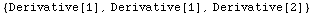Cases

Consider the list of (x,y,z) coordinates below.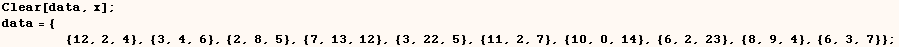The line below picks out all elements of data where the second of three in a list is greater than 7.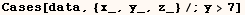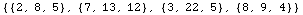Now the next line will pick the points where y>7 and return the list {x, y,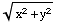}.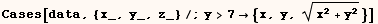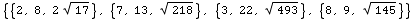The method above works as long as (x) doesn't have a global value.  In the cell below (x) has a global value and one doesn't get the expected result.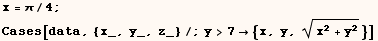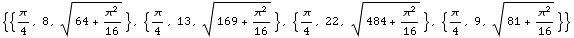The problem above can be avoided by using the line below. The difference is the use of (expr :→ x) instead of (expr → x).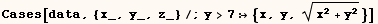Notice (x) still has a global variable.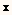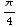In the previous examples involving Cases[data,{x_,y_,z_}....] all occurrences that met the pattern were returned.  Cases can take a fourth argument which indicates the maximum number of elements it should return.  In the next line the fourth argument is 1 so Cases only returns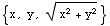for the first element where (y>7).  Notice that a third argument was necessary since we wanted to use the fourth argument.  In this example the third argument provided is the default level specification (1).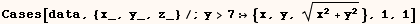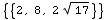In the previous examples Cases had only two arguments.  Cases can take a level specification as a third argument.  The default level specification is 1 or {1} (both are equivalent).  In the next line the default level specification is used, and Cases doesn't look inside each term of the sum.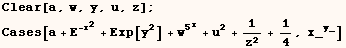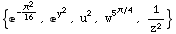The next example is from the online Help Browser that comes with Mathematica.  Here the level specification is {1,∞} and Cases looks at every level (except level 0).  As a result the list returned by Cases includes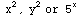.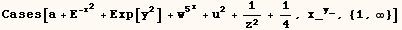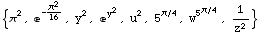I don't provide further examples, but any pattern matching constructs can be used in Cases. Nuances of pattern matching are discussed in another section.